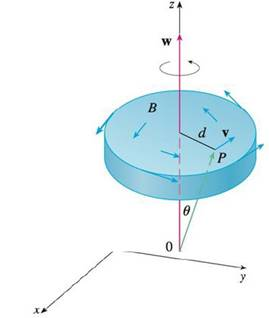Chapter 16.5, Problem 37E

Chapter
Section
Textbook Problem

This exercise demonstrates a connection between the curl vector and rotations. Let B be a rigid body rotating about the z-axis. The rotation can be described by the vector w = ωk, where ω is the angular speed of B, that is, the tangential speed of any point P in B divided by the distance d from the axis of rotation. Let r = ⟨x, y, z⟩ be the position vector of P.(a) By considering the angle θ in the figure, show that the velocity field of B is given by v = w × r.(b) Show that v = −ω y i + ω x j.(c) Show that curl v = 2w.(a)

To determine

To show: The vector v=w×r .

Explanation

Given data:

w=ωk and r=x,y,z .

ω=vd (1)

From Figure, sinθ=dr .

Rearrange the equation.

d=rsinθ

Substitute rsinθ for d in equation (1),

(b)

To determine

To show: The vector v=ωyi+ωxj .

(c)

To determine

To show: The curlv=2w .

Still sussing out bartleby?

Check out a sample textbook solution.

See a sample solution

The Solution to Your Study Problems

Bartleby provides explanations to thousands of textbook problems written by our experts, many with advanced degrees!

Get Started

Find more solutions based on key concepts